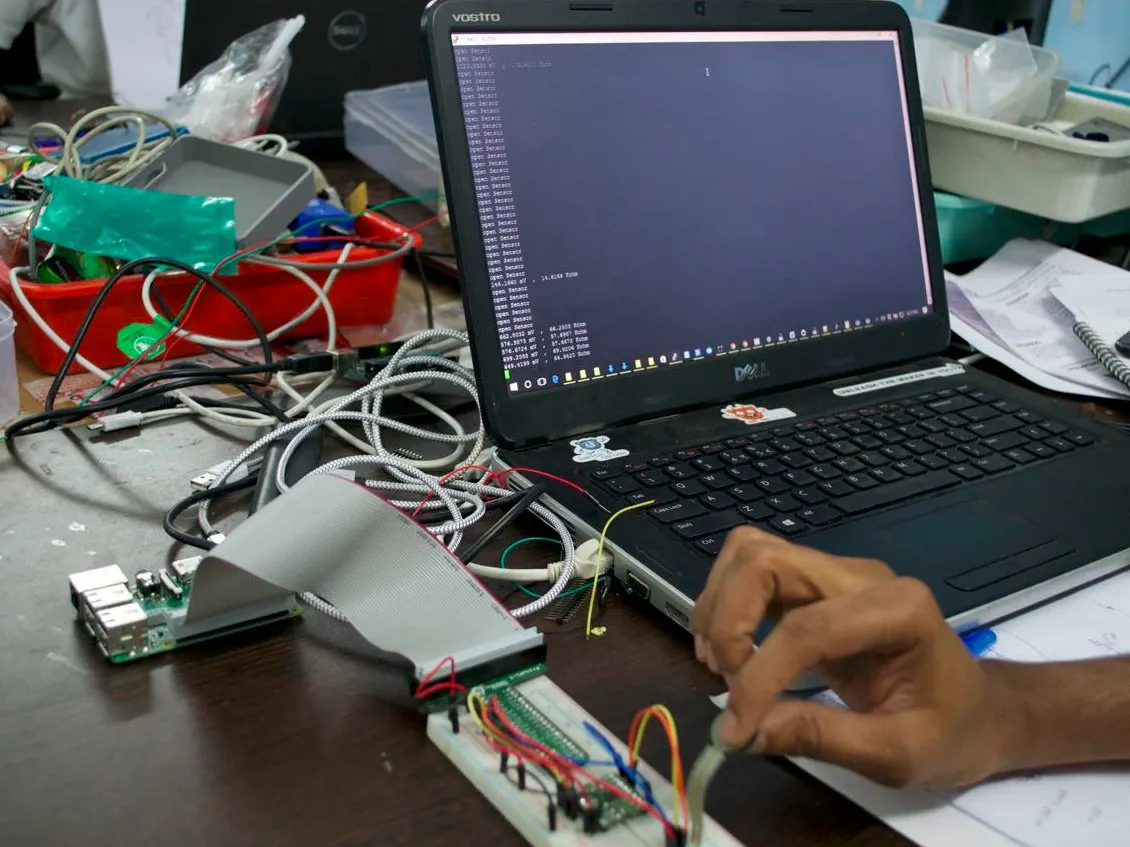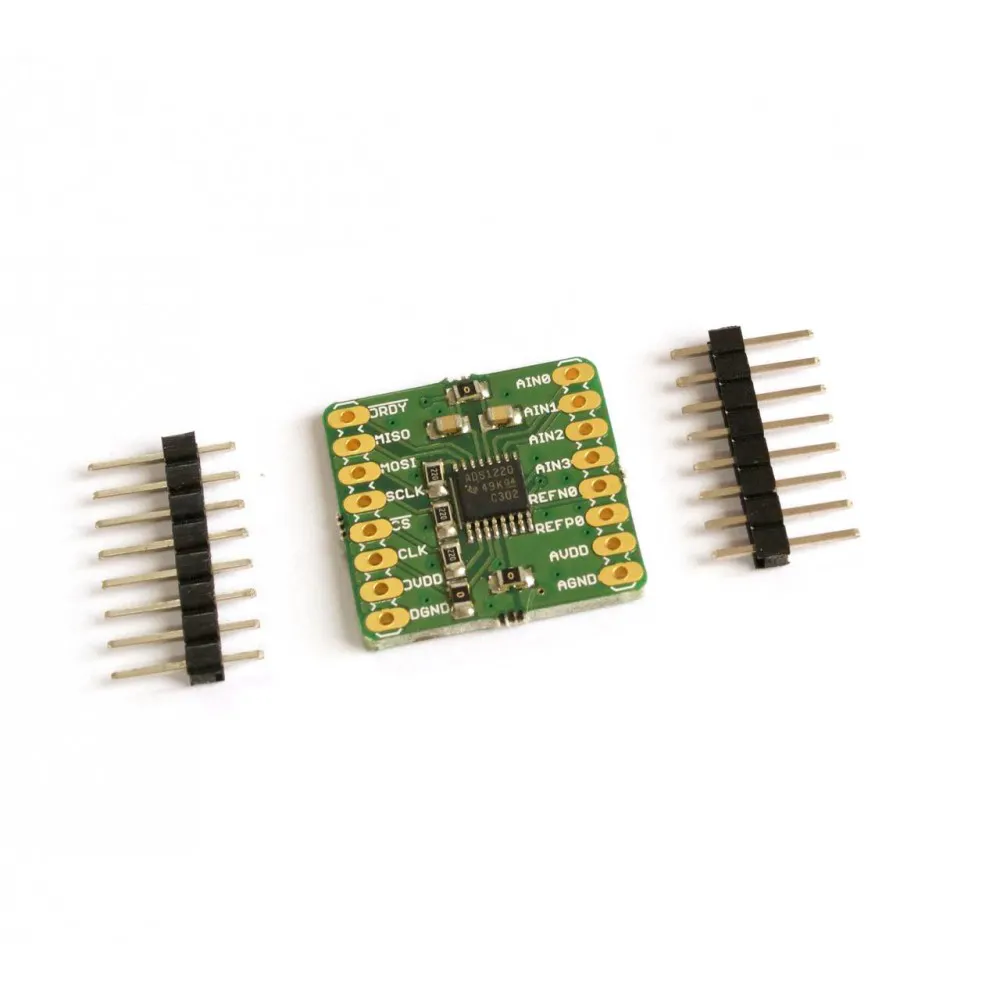# Resistive-sensor Measurement using Current Source

We show you how to excite a force-sensitive resistor using a current source, instead of the traditional Wheatstone bridge-based approach.

IntermediateProtip1 hour3,238## Things used in this project

### Hardware componentsRaspberry Pi 3 Model B
×1×1
 ProtoCentral PROTOPI-T V2 GPIO BREAKOUT WITH USB SERIAL ACCESS FOR RASPBERRY PI 3 / 2
×1

## Code

Python
```#!/usr/bin/env python
print "test"

import spidev
import time
import sys
import RPi.GPIO as GPIO
from array import *

RREG  =   0x20
WREG  =   0x40
START =   0x08
STOP  =   0x0A
RDATAC=   0x10
SDATAC	=	0x11
RDATA	=	0x12

GPIO.setmode(GPIO.BOARD)

# Pin declararion
DRDY_PIN  = 13
START_PIN = 15
CS_PIN    = 18
RESET_PIN = 16

GPIO.setwarnings(False);

#Set pin direction
GPIO.setup(CS_PIN,GPIO.OUT)
GPIO.setup(DRDY_PIN,GPIO.IN)

spi = spidev.SpiDev()				# create spi object
spi.open(0,1)					# open spi port 0, device (CS)1
spi.max_speed_hz = (500000)
spi.mode = (1)

print "test"
count = 0

def Reg_Write(address,data):    # write Fibonacci series up to
print ("Writing %s to Register %s" %( hex(data),hex(address)))
print(data);

GPIO.output(CS_PIN, False)
time.sleep(0.002)               # sleep for 0.1 seconds
GPIO.output(CS_PIN, True)
time.sleep(0.002)               # sleep for 0.1 seconds

GPIO.output(CS_PIN, False)
time.sleep(0.002)               # sleep for 0.1 seconds
resp = spi.xfer2([opcode1])        # transfer one byte
resp = spi.xfer2([data])        # transfer one byte
time.sleep(0.002)               # sleep for 0.1 seconds
GPIO.output(CS_PIN, True)
return;

GPIO.output(CS_PIN, False)
time.sleep(0.002)               # sleep for 0.1 seconds
GPIO.output(CS_PIN, True)
time.sleep(0.002)               # sleep for 0.1 seconds

GPIO.output(CS_PIN, False)
time.sleep(0.002)               # sleep for 0.1 seconds
resp = spi.xfer2([opcode1])        # transfer one byte
resp = spi.xfer2([0xff])        # transfer one byt
time.sleep(0.002)               # sleep for 0.1 seconds
GPIO.output(CS_PIN, True)

print(resp);
return;

def Spi_command(command):    # write Fibonacci series up to
GPIO.output(CS_PIN, False)
time.sleep(0.002)               # sleep for 0.1 seconds
GPIO.output(CS_PIN, True)
time.sleep(0.002)               # sleep for 0.1 seconds

GPIO.output(CS_PIN, False)
time.sleep(0.002)               # sleep for 0.1 seconds
resp = spi.xfer2([command])        # transfer one byte
time.sleep(0.002)               # sleep for 0.1 seconds
GPIO.output(CS_PIN, True)

return;

def initialisation1():
print ("INITIALISATION");
#GPIO.output(CS_PIN, False)

Spi_command(START);
time.sleep(0.1);
Spi_command(STOP);
time.sleep(0.1);

Spi_command(SDATAC);
time.sleep(0.3);

Reg_Write(0x00, 0x01);
time.sleep(0.01);
Reg_Write(0x01, 0x04);
time.sleep(0.01);
Reg_Write(0x02, 0x10);
time.sleep(0.01);
Reg_Write(0x03, 0x00);
time.sleep(0.01);

time.sleep(0.1);
time.sleep(0.1);
time.sleep(0.1);

Spi_command(RDATAC);
time.sleep(0.3);

Spi_command(START);
time.sleep(0.1);

GPIO.output(CS_PIN, True)
return;

def toHex(dec):
x = (dec % 16)
digits = "0123456789ABCDEF"
rest = dec / 16
if (rest == 0):
return digits[x]

def initialisation2():

time.sleep(0.2)
print "STARTED"

#GPIO.output(CS_PIN, False)
resp = spi.xfer2([0x11])        # transfer one byte
time.sleep(0.3)
return;

buff = [0,0,0,0,0,0,0,0,0];
GPIO.output(CS_PIN, False)
buff = spi.xfer2([0xff,0xff,0xff])
GPIO.output(CS_PIN, True)

print("0x %x %x %x\t"%(buff,buff,buff))
value = buff<<16 | buff<<8 | buff
print(value)
volt = (float(value) * .000244140625)
print  volt,"mV"
return buff;
#return r

return result

voltage = 0

#global voltage
while(GPIO.input(DRDY_PIN) == True):
time.sleep(0.001)

if (GPIO.input(DRDY_PIN) == False):
#if 1:
#val = ((data&0xff) << 16) | ((data&0xff) << 8) | (data&0xff)
val = ((data&0xff) << 16) + ((data&0xff) << 8) + (data&0xff)
#val = (data&0xff0000) + (data&0xff00) + (data&0xff)
val = int(val)

if( (val&(1<<(24-1))) != 0 ):
value = val - 0x1000000
else:
value = val

voltage = (float(value)*100/8388607)
return voltage

while True:

time.sleep(0.5)
```

### How to install and use this code

Plain text
```Install Python Libraries

To install Python packages:
Open the terminal type:
sudo apt-get update

sudo apt-get install python-dev
cd py-spidev
sudo make install

To install raspberry spi module
mkdir python-spi
cd python-spi
wget https://raw.github.com/doceme/py-spidev/master/setup.py
wget https://raw.github.com/doceme/py-spidev/master/spidev_module.c
sudo python setup.py install

To install raspberry gpio module
sudo apt-get install python-dev
sudo apt-get install python-rpi.gpio

Install time package
pip install time

Note:  Make Sure is serial interface is SPI is enabled.
Check Menu-> Preferences-> Raspberry Pi configuration -> SPI-> Enable
Connections

Pin1 (3.3V) -----> VDD
Pin9 (GND) -----> GND
Pin13 -------> DRDY
Pin 15 -------> START
Pin16 -------> RESET
Pin 18 ------->CS
Pin19 -------> MOSI
Pin 21 -------> MISO
Pin23 -------> SCK
Running script
Copy the example code “example.py” to “python-spi “ created.

Run the script using “sudo python example.py”

Code explanation

Reads the ADC value and convert it to corresponding voltage to be plotted

initialisation1()
Intialises the ads1292 to be in a Continuous conversion mode, 125SPS .

Writes one register to an address

Spi_command(command)
Sends a SPI command to the ads1292

generator()
Copies the ADC valus to an array, and send it to the plotter to be plotted
```

## Credits

### Ashwin K Whitchurch

12 projects • 79 followers